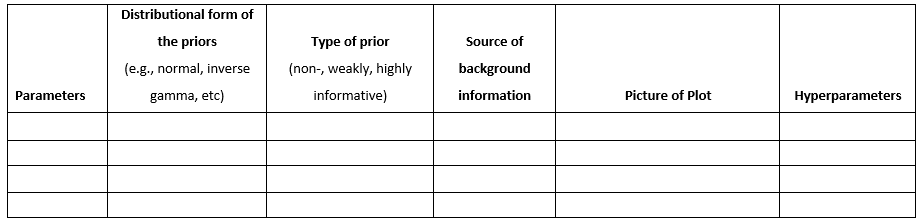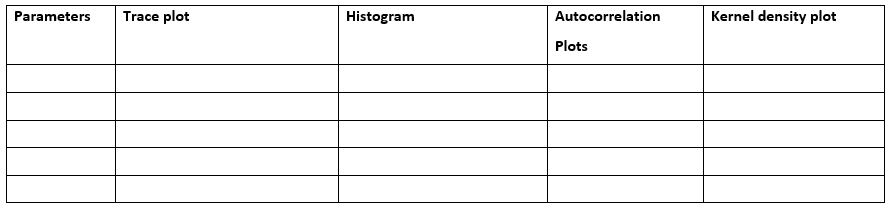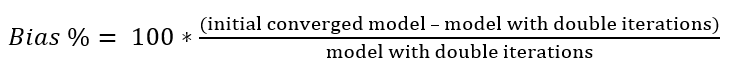# WAMBS-Checklist: RJAGS

## Solutions

Developed by Naomi Schalken and Rens van de Schoot

### Contents

Exercise 1 - WAMBS checklist
Example data 2

The WAMBS Checklis
When to worry, and how to Avoid the Misuse of Bayesian Statistics

To be checked before estimating the Model

To be checked after estimation but before inspecting Model
Step 1: Do you understand the priors?
Step 2: run the model and check for convergence
Step 3: doubling the number of iterations and convergence diagnostics
Step 4: Does the posterior distribution histogram have enough precision?
Step 5: Do the chains exhibit a strong degree of autocorrelation
Step 6: Does the posterior distribution make substantive sense?
Step 7: Do different specification of the multivariate variance priors influence the results?
Step 8: Is there a notable effect of the prior when compared with non-informative priors?
Step 9: Are the results stable from a sensitivity analysis?
Step 10: Is the Bayesian way of interpreting and reporting model results used?

### Exercise 1

In this exercise we will be following the first eight steps of the When-to-Worry-and-How-to-Avoid-the-Misuse-of-Bayesian-Statistics - checklist, or the WAMBS-checklist (Depaoli and van de Schoot 2015), to analyze data of PhD-students.

#### Example Data

The model we will be using for the first exercise is based on a study about predicting PhD-delays (Van de Schoot, Yerkes, Mouw and Sonneveld 2013). Among many other questions, the researchers asked the Ph.D. recipients how long it took them to finish their Ph.D. thesis (n=304). It appeared that Ph.D. recipients took an average of 59.8 months (five years and four months) to complete their Ph.D. trajectory. For the current exercise we will answer the question why some Ph.D. recipients took longer than other Ph.D. recipients by using age as a predictor (M = 30.7, SD = 4.48, min-max = 26-69). The relation between completion time and age is expected to be non-linear. It is expected that at a certain point in your life (i.e., mid-thirties), family life takes up more of your time than when you are in your twenties or when you are older. However, we expect that if you are in your mid-thirties and you are doing a Ph.D. you also take this extra time into account. The researchers asked Ph.D. candidates about their planned graduation day according to the original contract and their actual graduation day. The average gap between the two data appeared to be 9.6 months (SD = 14.4, min-max = -3 to 69). We expect that the lag between planned and actual time spent on the trajectory is less prone to non-linearity compared to actual project time.

So, in our model the GAP is the dependent variable and AGE and AGE2 are the predictors. The data can be found in the file `data_example.txt`.

#### Step 1: Do you understand the priors?

Before actually looking at the data we first need to think about the prior distributions and hyperparameters for our model. For the current model, we need to think about 4 priors:

• the intercept
• the two regression parameters (beta1 for the relation with AGE and beta2 for the relation with AGE2)
• and the residual variance (e)

Then, we need to determine which distribution to use for the priors. Let’s use for the

• intercept a normal prior with N(μ0, τ0), where μ0 is the prior mean of the distribution and τ0 is the precision parameter
• β1 a normal prior with N(μ0, τ0)
• β2 a normal prior with N(μ0, τ0)
• e an Inverse Gamma distribution with IG(κ0,θ0), where θ0 is the shape parameter of the distribution and κ0 the rate parameter

Note: In Bugs/Jags they use tau, τ (= precision), instead of sigma^2, σ2 (= variance) or sigma σ (= SD). If you have information about the variance (σ2) then you take the inverse by taking the quotient: 1/σ2. For example, if you want a prior variance of 100 in Jags, you do 1/variance = 1/100 = 0.01.

Next, we need to specify actual values for the hyperparameters of the prior distributions. Let’s say we found a previous study and based on this study the following hyperparameters can be specified:

• intercept ~ N(10,5)
• β1 ~ N(.8,5)
• β2 ~ N(0,10)
• ∈ ~ IG(0.5, 0.5)

To make some nice plots of the prior distribution we created a function in R. First copy paste the following syntax into R (without making any changes):

``````prior.plot <- function(type="normal", mean=0, variance=100, shape=0, scale=1, sec.min=-50, sec.max=50, step=.1, label=label) {
x <- seq(sec.min, sec.max, by = step)
if (type == "normal") {
prior.d <- dnorm(x,mean = mean, sd = sqrt(variance))
}
if (type == "gamma") {
prior.d <- dgamma(x,shape = shape, scale = scale)
}
``````  plot(x, prior.d, type="l", xlab = label)
abline(v = mean, col="red")
abline(v = (mean+2*sqrt(variance)), col = "blue")
abline(v = (mean-2*sqrt(variance)), col = "blue")
}``````

Input:

• Type = either “normal” or “gamma”
• Mean = the mean of the distribution
• Variance = the variance of the distribution
• Shape = for gamma: the shape of the distribution
• Scale = for gamma: the scale of the distribution
• sec.min/.max and step = make the interval shown on the x-axis smaller or wider and take smaller or larger steps
• label = label of the x-axis

For example, to obtain a plot for the intercept with a normal prior with a mean of 10 and a variance of 100, use the syntax:

``prior.plot(type = "normal", mean = 10, variance = 100, label="intercept")``

To plot all four priors use this code, but fill in values for X:

``````par(mfrow=c(2,2))
prior.plot(type = "normal", mean = X, variance = X, label="intercept")
prior.plot(type = "normal", mean = X, variance = X, label="beta 1")
prior.plot(type = "normal", mean = X, variance = X, label="beta 2")
prior.plot(type = "gamma",  shape = X, scale = X, sec.min=0, sec.max=0.5, step=.001, label="res.var")
par(mfrow=c(1,1))``````

Now you can fill in the first table of the WAMBS-checklist (see the next page):#### Step 2: run the model and check for convergence

Load the data and make sure you set the working directory to the folder where your data is stored.

To set your working directory to the right folder use:

``setwd("C:/your/folder/here")``

For example:

``setwd("E:/exercise-day-4")``

To load the data

``data <- read.table("data_example.dat")``

Variables in the model:

• y1 = project time
• y2 = lag between planned PhD duration and actual PhD duration
• x1 = age of participant (centered)
• x2 = age squared

To make the code we will use later a bit easier, we split the data into vectors for each variable:

``````y1 <- data[,1]
y2 <- data[,2]
x1 <- data[,3]
x2 <- data[,4]``````

Specify number of iterations and number of burn-in iterations (that will not be included for estimating the posterior):

``````iterations <- 200
burnin <- floor(iterations/2)
chains <- 2``````

A general way of deciding on the amount of burn-in iterations is to use half of your total number of iterations as burn-in.

Create a text file that contains the model you want to analyze the data with using, for example, notepad. Do not forget to change the labels in red (make sure to use the precision for the prior variance - to go from variance to precision do 1/variance = precision):

``````model
{
for (i in 1: N )
{y[i] ~ dnorm(mu[i], tau)}
for (i in 1: N )
{ mu[i] <-  intercept + beta1 * x1[i] + beta2 * x2[i] }
intercept ~ dnorm( X , X )
beta1 ~ dnorm( X , X ) beta2 ~ dnorm( X , X )
tau   ~ dgamma( X , X )
````sigma2 <- inverse(tau) } ````

Save this file with a .txt extension.

Now, run RJAGS to initialize the model:

``````model.fit <- jags.model(file="FILE NAME.txt",
data=list(y=y2, x1=x1, x2=x2), n.chains = chains)``````

where the txt file holds the Bugs model input (should be saved in your working directory) and data is a named list of the vectors you specified above. The labels on the left-hand side of the = are the labels used in your model txt file, the labels on the right-hand side of the = are the vectors in which you have stored the actual data.

Run the model for a certain number of MCMC iterations and extract random samples from the posterior distribution of parameters of interest. First, specify the model object and then the parameters you would like to have information on. N.iter is the number of iterations to monitor. We specified this number above.

``````model.samples <- coda.samples(model.fit, c("intercept", "beta1", "beta2",
"sigma2"), n.iter=iterations)``````

To obtain summary estimates of the parameters use

``summary(window(model.samples, start = burnin))``

To obtain diagnostic plots to check convergence for all parameters use

``plot(model.samples, trace=TRUE,  density = FALSE)``

Now, you can fill in columns 1 and 2 of Table 2 (the information for the other columns will be added later in the exercise):#### Step 3: doubling the number of iterations and convergence diagnostics

To obtain convergence diagnostics to check convergence for all parameters we use the Gelman and Rubin diagnostic (Gelman and Rubin 1992) and the Geweke (1992) diagnostic. To obtain the G-R diagnostic use

``````gelman.diag(model.samples)
gelman.plot(model.samples)``````

Look at the Upper CI/Upper limit this should be close to 1. When you add autoburnin=FALSE, the burn-in phase of the chain length will be included in the #calculation, resulting (normally) in a higher PSR value.

To obtain the Geweke diagnostic use

``geweke.diag(model.samples)``

This syntax will provide a z-score, where a z-score higher than the absolute value of 1.96 is associated with a p-value of < .05 (two-tailed). These values should therefore be lower than the absolute value of 1.96.

If Geweke indicates that the first and last part of a sample from a Markov chain are not drawn from the same distribution, it may be useful to discard the first few iterations to see if the rest of the chain has “converged”. This plot shows what happens to Geweke’s z-score when successively larger numbers of iterations are discarded from the beginning of the chain.

``geweke.plot(model.samples)``

Did the model reach convergence?

We want to be absolutely sure the model reached convergence and therefore we re-run the model with twice the number of iterations and compute the relative bias .

````
``````iterations <- X
burnin <- floor(iterations/2)``````

… and initialize/run the model with the following names:

``````model.fit.2 <- jags.model(...)
model.samples.2 <- coda.samples(...)``````

Although you could compute the bias by hand, I created an easy function to quickly compute bias of parameter estimates between two models. First copy past the following syntax into R (without making any changes):

``````bias.model <- function(model1, model2) {
bias <- as.vector(1)
for (i in 1:nrow(model1[])) {
bias[i] <- (model2[][i,1]-model1[][i,1])/model1[][i,1]*100
}
bias <- cbind(bias, as.vector(model1[][,1]), as.vector(model2[][,1]))
rownames(bias) <- row.names(model1[])
colnames(bias) <- c("% bias", "est model 1", "est model 2")
print(bias)
}``````

To compare the two models first retrieve the summary statistics from the models you want to compare (start indicates the first iteration you would like to include, usually the iterations after the burn-in phase).

``````sum.model.1 <- summary(window(model.samples, start = 100))
sum.model.2 <- summary(window(model.samples.2, start = 250))
}``````

Then use

``bias.model(sum.model.1, sum.model.2)``

Did the model reach convergence, or do you want to re-run the model with even more iterations? Continue until you are satisfied.

#### Step 4: Does the posterior distribution histogram have enough precision?

To check the histograms use the following code:

``````par(mfrow=c(2,2))
hist(model.samples.2[][,1], breaks = 50, main = "Intercept")
hist(model.samples.2[][,2], breaks = 50, main = "Age")
hist(model.samples.2[][,3], breaks = 50, main = "Age squared")
hist(model.samples.2[][,4], breaks = 50, main = "Residual variance")
par(mfrow=c(1,1))``````

Copy-paste the histograms to Table 2. What is your conclusion; do we need even more iterations?

#### Step 5: Do the chains exhibit a strong degree of autocorrelation

To check the autocorrelation plots use the following code (make sure to refer to your final model.samples file):

``autocorr.plot(model.samples.2)``

Copy-paste the plots to Table 2. What is your conclusion; do we need even more iterations?

#### Step 6: Does the posterior distribution make substantive sense?

To check the Kernel density plots use the following code (make sure to refer to your final model.samples file):

``plot(model.samples.2, trace=FALSE,  density = TRUE)``

Copy-paste the plots to Table 2. What is your conclusion; do the posterior distributions make sense?

#### Step 7: Do different specification of the multivariate variance priors influence the results?

To understand how the prior on the residual variance impacts the results, we compare the previous model with a model where different hyperparameters for the Gamma distribution are specified.

The specification of the hyperparameters .5, .5 was inspired by this paper (Van de Schoot, Broere, Perryck, Zondervan-Zwijnenburg and Van Loey 2015) but appears to be a very informative prior. A non-informative specification would be to use .001, .001 (Asparouhov and Muthén 2010).

Specify number of iterations and number of burn-in iterations:

``````iterations <- X
burnin <- floor(iterations/2)``````

Create a file that contains the model you want to analyze the data using for example notepad and save this file with a .txt extension (for example model_example_gamma.txt). Initialize and run the model in JAGS:

``````model.fit.3 <- jags.model(file="model_example_gamma.txt", . )
model.samples.3 <- coda.samples(model.fit.3, . )
Save the output
sum.model.3 <- summary(model.samples.3, start = burnin)
request for the posterior results
sum.model.3
and compare the relative bias:
bias.model(sum.model.2, sum.model.3)``````

Are there any substantial differences?

#### Step 8: Is there a notable effect of the prior when compared with non-informative priors?

Specify number of iterations and number of burn-in iterations:

``````iterations <- X
burnin <- floor(iterations/2)``````

Create a file that contains the model you want to analyze the data using for example notepad and save this file with a .txt extension (for example model_example_noninf.txt). Initialize and run the model in JAGS:

``````model.fit.4 <- jags.model(file="model_example_noninf.txt", ... )
model.samples.4 <- coda.samples(model.fit.4, ... )``````

Did this non-informative model reach convergence (think about all the previous steps)?

If so, save the output;

``````sum.model.4 <- summary(model.samples.4, start = burnin)
request for the posterior results
sum.model.4
and compare the relative bias:
bias.model(sum.model.2, sum.model.4)``````

What is your conclusion about the influence of the priors on the posterior results?Which results will you use to draw conclusion on?

### Exercise 2

If you want to use Bayesian statistics in different software, you could take a look at our How to get started tutorials. Here you will find basic set-up files for 9 different software packages. Don’t try to run/understand all the different software packages today, but use the information instead as starting point if you want to use other software.

### References

Asparouhov, T., & Muthén, B. (2010). Bayesian analysis of latent variable models using Mplus. Retrieved June 17, 2014, from http://www.statmodel2.com/download/BayesAdvantages18.pdf.

Depaoli, S., and van de Schoot, R. (2015). Improving transparency and replication in Bayesian Statistics: The WAMBS-Checklist. Psychological Methods.

Gelman, A and Rubin, DB (1992) Inference from iterative simulation using multiple sequences, Statistical Science, 7, 457-511

Van de Schoot, R., Broere, J.J., Perryck, K., Zondervan-Zwijnenburg, M., & Van Loey, N. (2015). Analyzing Small Data Sets using Bayesian Estimation: The case of posttraumatic stress symptoms following mechanical ventilation in burn survivors. European Journal of Psychotraumatology.

Van de Schoot, R., Yerkes, M., Mouw, J. & Sonneveld, H. (2013). What took them so long? Explaining PhD delays among Doctoral Candidates. PLoS One, 8(7), e68839. doi: 10.1371/journal.pone.0068839.

### Other tutorials you might be interested in

The WAMBS-Checklist

MPLUS: How to avoid and when to worry about the misues of Bayesian Statistics

Blavaan: How to avoid and when to worry about the misuse of Bayesian Statistics

-----------------------------------------------------------

First Bayesian Inference

FBI - MPLUS

FBI - Blavaan

FBI - RShiny

WordPress Theme built by Shufflehound. © Rens van de Schoot   All rights reserved   2019